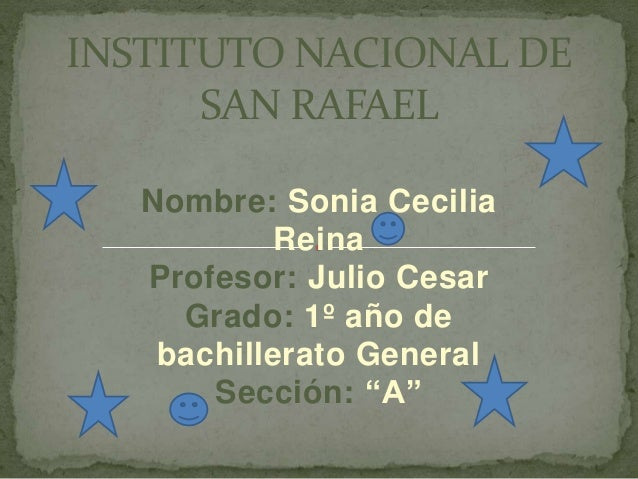Successfully reported this slideshow.
We use your LinkedIn profile and activity data to personalize ads and to show you more relevant ads. You can change your ad preferences anytime.Upcoming SlideShare
×

# Julio cesar

49 views

Published on

lo k kiera

Published in: Education
• Full Name
Comment goes here.

Are you sure you want to Yes No
Your message goes here• Be the first to comment

• Be the first to like this

### Julio cesar

1. 1. Nombre: Sonia Cecilia Reina Profesor: Julio Cesar Grado: 1º año de bachillerato General Sección: “A” INSTITUTO NACIONAL DE SAN RAFAEL
2. 2. CONCENTRACIONES FISICAS Y QUIMICAS DE LAS SOLUCIONES.
3. 3.  Las unidades físicas de concentración de las soluciones tienen que ver con la proporción entre las partes del soluto respecto al volumen total de la solución ,esta se puede expresar como partes masa sobre masa, volumen sobre volumen, masa sobre volumen y partes por millón. UNIDADES FISICAS DE CONCENTRACION DE LAS SOLUCIONES.
4. 4. Formula: %m/m=peso del soluto/peso de la solución*100 Ejercicio Nº 1. Calcular el porcentaje en masa de una solución de sulfato de cobre, en agua si contiene 25g de soluto en 300g de disolución. %m/m=25g de sto/300g de sol * 100 %m/m=8.3% Porcentaje referido a la masa sobre masa (%m/m)
5. 5. Formula: %v/v=volumen del sto/volumen de la sol*100 Ejercicio Nº 2. Que porcentaje en volumen tendrá una solución, disolviendo 80ml de metanol en 800ml de la solución. %v/v=80ml/800ml*100 = 10% Porcentaje referido al volumen sobre volumen (%v/v)
6. 6. Formula: %m/v=gramos de sto/volumen de la sol * 100 Ejercicio Nº 3. Si tenemos 5g de cloruro de sodio y 250ml de la solución; ¿Cuál es el porcentaje masa volumen? %m/v=5g del sto/250ml de la solución*100 %m/v= 2% Porcentaje referido masa sobre volumen (%m/v)
7. 7. Formula: ppm=mg/kg ppm=mg/2 Se define como los miligramos (mg) del soluto contenidos en un kg o litro del solvente. Ejercicio Nº 4. Una muestra de agua de 500ml tiene 4 ml de flúor ¿Cuántas partes por millón de flúor hay en la muestra? Ppm=500ml/1,000L Ppm = 4ml/0.5= 8 partes por millón. Partes por millón (ppm)
8. 8. La concentración: se refiere a la cantidad de soluto que hay en una masa determinada de solución con solvente. Puesto que términos como concentrado, diluido saturado o insaturado son en especifico, existen maneras de expresar la cantidad de soluto de una solución. Las cuales son: MORALIDAD,MODALIDAD,NORMALIDAD. UNIDADES QUIMICAS DE CONCENTRACION DE LAS SOLUCIONES.
9. 9. La moralidad se refiere al numero de moles de soluto que están presentes por litro de soluciones. Por ejemplo: si una solución tiene una concentración de 2.5 molar, que significa que hay 2.5 moles de soluto por cada litro de solución. Es importante notar que el volumen de solvente no es tomado en cuenta si no el volumen final de la solución. Para tales efectos se presenta la siguiente formula general. Formula: Moralidad=moles de soluto/litros de la solución. M=moles de sto/L de la sol La moralidad
10. 10. Calcular la moralidad de una solución que contiene 32g de cloruro de sodio (NaCl) en 0.75L de una solución. PMG,NaCl moles sto=gtto/PGM Na=22.9898 =32g/58.4428 Cl=35.453 =0.54 moles/sto R// =58.4428g/mol =0.54mol/0.75L M=0.72m =0.72 M Ejercicio Nº 1
11. 11. Es el numero de moles de soluto contenidos en un kilogramo de solvente. Una solución formada por 36.5g de acido clorhídrico HCL y 1000g de agua es una solución a 1molal=1m Formula: moles de soluto/kg de solvente Molalidad
12. 12. Calcular la Molalidad de una solución que tiene 0.1 moles de cloruro de sodio en 0.2 kg de agua. M=0.1moles/0.2kg M=0.5 molal Ejercicio Nº 2
13. 13. Es el numero de equivalentes gramo de soluto contenidos en un litro de solución. Y su formula general es la siguiente. Formula: N=Nº de Eq-g de soluto/litros de solución. Equivalentes químicos. Un equivalente químico (EQ), también llamado peso equivalente o equivalentes gramos, es la cantidad de una sustancia que reacciona para producir un mol de producto. La normalidad
14. 14. Resuelva HCl. H=1.0079 =37.24 Cl=35.45 1 37.24 =37.24 Ejercicio Nº 3